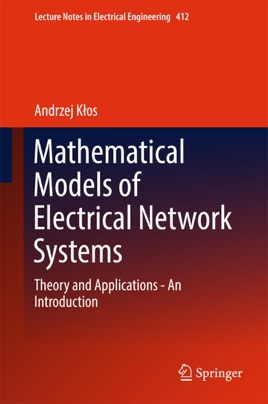• \$109.99

## Publisher Description

This book is for all those who are looking for a non-conventional mathematical model of electrical network systems. It presents a modern approach using linear algebra and derives various commonly unknown quantities and interrelations of network analysis. It also explores some applications of algebraic network model of and solves some examples of previously unsolved network problems in planning and operation of network systems. Complex mathematical aspects are illustrated and described in a way that is understandable for non-mathematicians. Discussing interesting concepts and practically useful methods of network analysis, it is a valuable resource for lecturers, students, engineers and research workers.